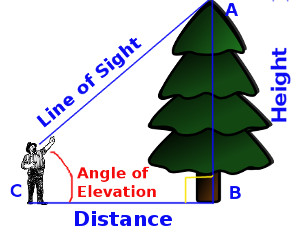Arithmetic
Arithmetic Progression
Associative Property
Averages
Brackets
Closure Property
Commutative Property
Conversion of Measurement Units
Cube Root
Decimal
Divisibility Principles
Equality
Exponents
Factors
Fractions
Fundamental Operations
H.C.F / G.C.D
Integers
L.C.M
Multiples
Multiplicative Identity
Multiplicative Inverse
Numbers
Percentages
Profit and Loss
Ratio and Proportion
Simple Interest
Square Root
Unitary Method
Algebra
Cartesian System
Order Relation
Polynomials
Probability
Standard Identities & their applications
Transpose
Geometry
Basic Geometrical Terms
Circle
Curves
Angles
Define Line, Line Segment and Rays
Non-Collinear Points
Parallelogram
Rectangle
Rhombus
Square
Three dimensional object
Trapezium
Triangle
Trigonometry
Trigonometry Ratios
Data-Handling
Arithmetic Mean
Frequency Distribution Table
Graphs
Median
Mode
Range

Solved Problems
Home >> Trigonometry Ratios >> Find Height, Distance using T - Ratios >> Angle of Elevation >>

## Angle of Elevation and find Height & Distance

 Angle of Elevation Angle of Depression

Before you study this topic you should know what is angle of elevation and depression:

What is Angle of Elevation ?

The formulas we used in angle of elevation :

To find height and distance we use Tan θ = Opposite Side / Adjacent Side
To find length or hypotenuse we use Sin θ = Opposite Side / Hypotenuse

Example 1 : A person is standing 5m away from tree, the angle of elevation of the top of tree is 60° find the height of tree ?
Solution : Let AB is the tree. B is the foot and A is the top of tree. C is the point on the ground where the person is standing and which is making the angle of elevation of 60°

If we draw a picture it will look like as below -so we get
CB (Distance from tree) = 5m
∠BCA = 60°
Right angle is at point B
AB (Height) = ?

A right angle triangle △ ABC is formed in which
AB is the Opposite Side from angle of elevation
BC is the Adjacent Side from angle of elevation

We know that Tan θ = Opposite Side / Adjacent Side

 tan 60° = ABBC

 √ 3 = AB10

 AB = 10 x √ 3

Value of  3  is 1.73 so,

AB = 5 x 1.73 = 8.65

AB = 8.65m is the height of tree

Example 2 :Height of tree is 8.65m, the angle of elevation of the top of tree is 60° find the distance at which the person is standing away from tree ?
Solution : Let AB is the tree. B is the foot and A is the top of tree. C is the point on the ground where the person is standing and which is making the angle of elevation of 60°

If we draw a picture it will look like as below -so we get
CB (Distance from tree) = ?
∠BCA = 60°
Right angle is at point B
AB (Height) = 8.65m

A right angle triangle △ ABC is formed in which
AB is the Opposite Side from angle of elevation
BC is the Adjacent Side from angle of elevation

We know that Tan θ = Opposite Side / Adjacent Side

 tan 60° = ABBC

 √ 3 = AB10

 AB = 10 x √ 3

Value of  3  is 1.73 so,

8.65 = CB x 1.73
CB = 8.65 / 1.73
CB = 5

so the person is standing 5m away from the tree

Example 3 : A tree is 5m high. If angle of elevation is 45° find the hypotenuse

Let AB is the tree. B is the foot and A is the top of tree. C is the point on the ground where a person is standing and which the angle of elevation of 45°

If we draw a picture it will look like as below -so we get
AC = ?
∠ BCA = 45°
AB = 5m

A right angle triangle △ ABC is formed in which
AB is the Opposite Side from angle of elevation
AC is the Hypotenuse Side

We know that Sin θ = Opposite Side / Hypotenuse

 sin 45° = ABAC

 1   √ 2 = 5AC

AC = 5 x  2

Value of  2  is 1.41 so,

5 x 1.41 = 7.05

AC = 7.05m is the hypotenuse

### Study More Solved Questions / Examples

Copyright@2020 Algebraden.com (Math, Algebra & Geometry tutorials for school and home education)